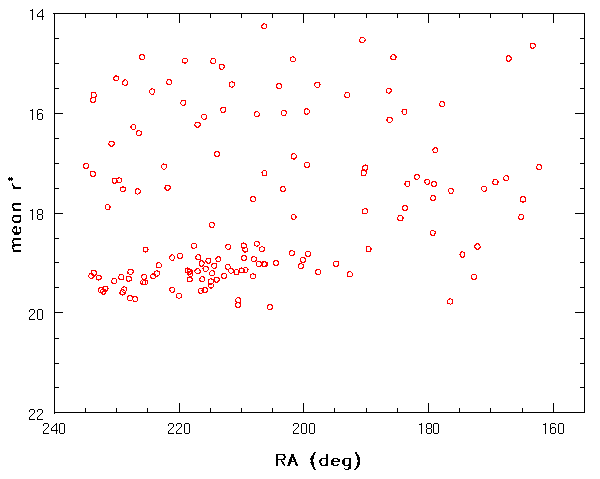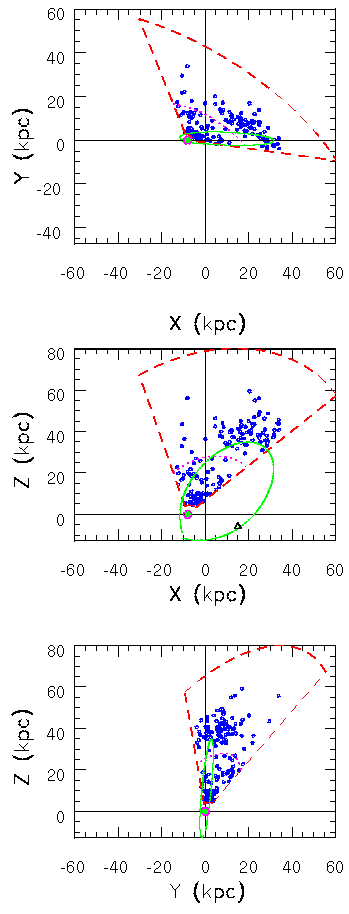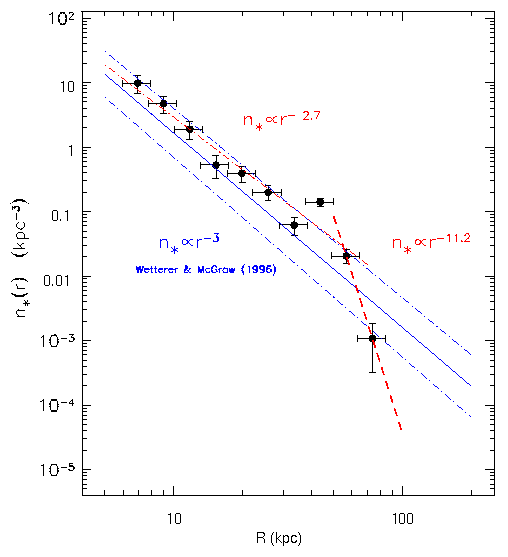Candidate RR Lyrae Stars Found in SDSS Commissioning Data

The following figure shows the distribution of candidate RR Lyrae stars, marked as red open circles, in the mean r vs. RA diagram. The data were obtained in six parallel scanlines, each 13.7 arcmin wide, along the Celestial Equator (-1.2687 < Dec < 1.2676). Note the concentration of sources with r=19-19.5 and 205 < RA < 230.The following figure shows the Galactic distribution of candidate RR Lyrae stars, displayed as blue circles (the large green circle marks the Sun's position at X=-8 kpc, Y=0, Z=0). The dashed lines show the volume within which our data can detect RR Lyrae stars: a very thin wedge with an opening angle of 80 deg and distances ranging from 5 kpc (saturation limit, r = 14) to 90 kpc (faint limit, r = 21). The dotted lines show the intersection of this wedge with a galactocentric sphere of radius 30 kpc (r = 18-18.5).The clump of stars centered on (X=20 kpc, Y=10 kpc, Z=40kpc), corresponds to the concentration of sources with r=19-19.5 and 205 < RA < 230 visible in the first figure. The clump center is in a similar direction (l=340, b=60) as the center of the Sagittarius dwarf spheroidal galaxy at (l=5.6, b=-14.0). The Sagittarius dwarf spheroidal is the closest known Galactic satellite, with a galactocentric distance of 16 kpc (in the above figure it is situated at X=15 kpc, Y=-2 kpc, Z=-6 kpc and marked by a triangle in the middle panel). The distance between its center and the clump is about 50 kpc. This is significantly larger than the extent of either structure (about 10 kpc) and probably implies that they are not physically associated. It is interesting, however, that the Galactic orbit of the Sgr dwarf spheroidal, as calculated by both Ibata et al. (1997) and Johnston et al. (1999) crosses the clump of candidate RR Lyrae stars. This orbit, taken from Johnston et al., is displayed by a solid line (the direction of Sgr dwarf's motion is towards the clump). Such close proximity between the calculated orbit and the clump of candidate RR Lyrae stars may perhaps be evidence for presumed debris caused by tidal disruption of the Sgr dwarf spheroidal in the Galactic potential.

The following figure shows the comparision between the volume density for RR Lyrae stars obtained from our data (dots with error bars) and the 1/R^3 power law determined by Wetterer & McGraw (1996, thick solid line with the 1 sigma uncertainty shown by thin dot-dashed lines) from data with R < 30 kpc. We find that the volume density follows a shallower power law with the best-fit power index of 2.7 +- 0.2 (thick dot-dashed line) for R < 50 kpc, and turns off sharply as a rather steep power law for R > 60 kpc (thick dashed line). However, Data for R < 35 kpc are consistent with the 1/R^3 distribution.These results are taken from a paper submitted to Astronomical Journal in January 2000. The reference is Ivezic, Z., Goldston, J., Finlator, K. et al. (for the SDSS Collaboration), 2000, AJ, submitted.